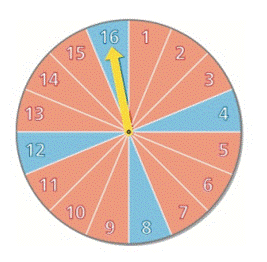Chapter 14.2, Problem 30E### Algebra and Trigonometry (MindTap ...

4th Edition
James Stewart + 2 others
ISBN: 9781305071742

#### Solutions

Chapter
Section### Algebra and Trigonometry (MindTap ...

4th Edition
James Stewart + 2 others
ISBN: 9781305071742
Textbook Problem

# 2 9 - 3 0 Refer to the spinner in Exercises 21 − 22 .Find the probability that the spinner has stopped on a number divisible by 3 , given that it has stopped on blue. 2 1 - 2 2 Refer to the spinner shown in the figure. Find the probability of the given event.(a) The spinner stops on red.(b) The spinner stops on an even number.(c) The spinner stops on red or an even number.(a) The spinner stops on blue.(b) The spinner stops on an odd number.(c) The spinner stops on blue or an odd number

To determine

To find:

The conditional probability that the spinner has stopped on a number divisible by 3, given that it has stopped on blue.

Explanation

Given:

The spinner is shown below:

Approach:

p(A|B)=p(AB)p(B)(1)

Here, p(A|B) is the conditional probability of an event A given that B has already occurred, p(AB) is the joint probability of events A and B, p(B) is the probability of event B.

Calculation:

There are 16 numbers in the spinner out of which 8 are even and 8 are odd numbers.

There are 12 red portions and 4 blue portions in the spinner.

Suppose E is the event of number divisible by 3 and B is the event of blue portion.

p(E)=516

Here, p(E) is the probability of a number divisible by three on spinner.

p(B)=416

Here, p(B) is the probability of blue portion on the spinner

### Still sussing out bartleby?

Check out a sample textbook solution.

See a sample solution

#### The Solution to Your Study Problems

Bartleby provides explanations to thousands of textbook problems written by our experts, many with advanced degrees!

Get Started